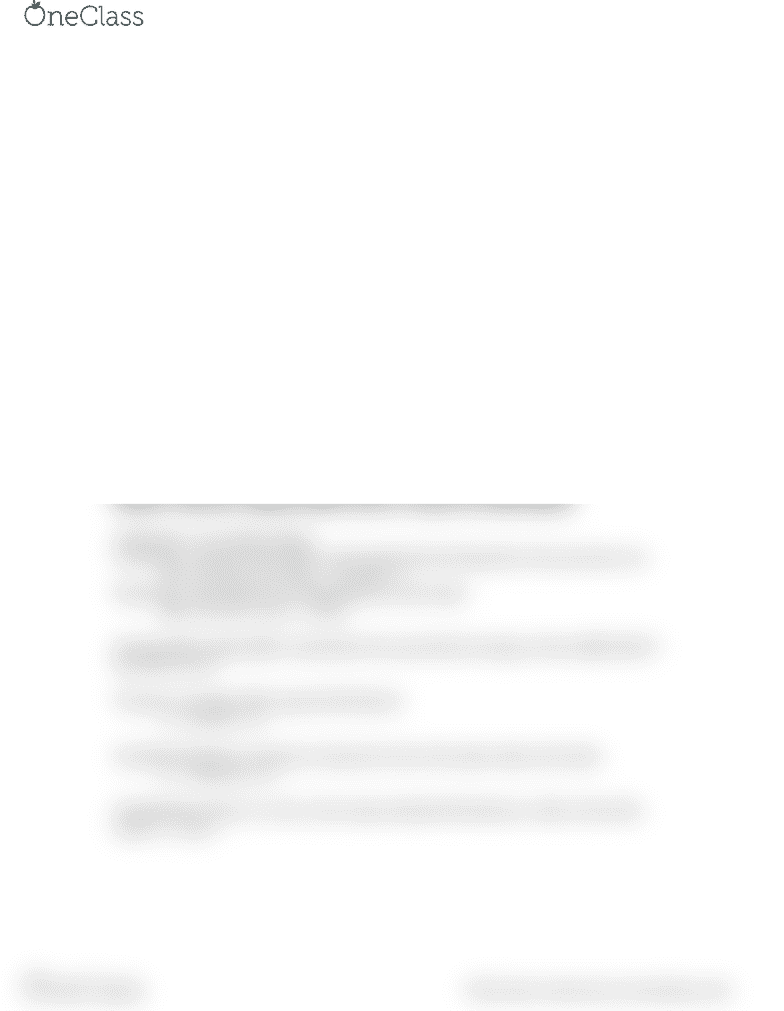Textbook Notes (280,000)
CA (170,000)
UBC (3,000)
SPAN (10)
Chapter 5

# SPAN 101 Chapter Notes - Chapter 5: Squared Deviations From The Mean, Interquartile Range, Standard Deviation

Department
Spanish
Course Code
SPAN 101
Professor
Enrique Manchon
Chapter
5

This preview shows half of the first page. to view the full 1 pages of the document.CH 5 DISPLAYING AND DESCRIBING QUANTITATIVE DATA
If the shape is skewed:
report the median and IQR
mean + standard deviation (mean and median differ)
If the shape is unimodal and symmetric:
mean and standard devation
possibly the median and IQR
unimodal: IQR is usually a bit larger than the standard deviation
Median + IQR; mean + standard deviation
Boxplots
Centre: a box that shows the middle half of the data
Height of the box: IQR
Upper fence = Q3 + 1.5 IQR
Lower fence = Q1 1.5 IQR
Whiskers: skewness
Average: ý = Total / n = Ey/n
Median: resistant to unusual observations or shape of the distribution
Arithmetic: non related values
Geometric: used for rates where each measurement depends on the previous one.
GM: √+R x +R x… x+Rn - 1
Harmonic: rates that are not dependent on each other
(M: n//y+/y+…/yn
Interquartile range (IQR): summarizes the spread by focusing on the middle half of
the data. Q3-Q1
Variance: average of the squared deviations
s2 = E(y-ý)2/ n-1
Standard deviation: measures of spread to have the same units as the data
s2 = E(y-ý)2/ n-1
Standardized value or z score: how many standard deviations a value is from the
mean: z = y-ý/s
find more resources at oneclass.com
find more resources at oneclass.com